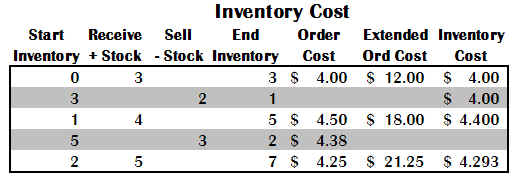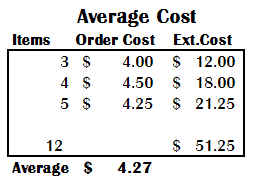Cost of Inventory Reports

As discussed in the Costs Topic earlier, XpertMart uses two different costs: Order Cost and Inventory Cost. Accordingly, the system provides different ways to calculate the cost of your inventory which are discussed below.

How XpertMart Calculates Inventory Cost
The majority of reports in XpertMart use Inventory Cost when calculating margin and profitability so it is important to understand how Inventory Cost is calculated. The following is an example, where the same items is received three different times at three different costs: \$4.00, \$4.50 and \$4.25.The first time three pieces are received at \$4.00 each. The Inventory Cost is \$4.00 (\$12.00/3 =\$4.00).  Following this first Receipt, two items are sold. For the purposes of calculating margin and profitability, the Inventory Cost is \$4.00. At this point, Inventory Cost and Order Cost are equal.

However, the second time this item is received, the Order Cost has changed to \$4.50. Four pieces are received at this new Order Cost of \$4.50. Now the Inventory Cost has changed because the Order Cost has been different the two times this item was received. The new Inventory Cost is \$4.40  because it is a weighted average ( (\$4.00*1) + (\$4.50*4) / 5) of the one piece already in stock (at a cost of \$4.00) and the four new pieces (at a cost of \$4.50 each).

Following this second Receipt, three pieces are sold, leaving two in stock. The third time the items is received the Order Cost has changed yet again to \$4.25. Five new pieces are received at this new Order Cost. The new Inventory Cost is \$4.293 because it is a weighted average ((\$4.40 * 2) + (\$4.25 * 5) / 7) of the two pieces already in stock (at a cost of \$4.40) and the five new pieces (at a cost of \$4.25 each). Note that the system will only display two decimal points but actually stores seven decimal points in the database. So while you might see \$4.29 on the screen, the database is actually storing \$4.2928571).

As this example shows, the Inventory Cost of this item (\$4.29) is different than the Order Cost of this item (\$4.25). The Inventory Cost reflects what you have historically paid to have the current items in stock and the Order Cost is merely the cost you would expect to pay on future orders.

There is yet another way to calculate costs and that is a simple weighted average. For any time period you care to analyze you can simply add up the items added to stock at the cost they were received and divide the total by the number of items. In the example discussed, a total of 12 items were received at a total cost of \$51.25, yielding an average cost of \$4.27.This Average Cost method is not as dynamic as Inventory Cost because it doesn't take into account the length of time merchandise sits in inventory before it sells. Also, it is somewhat arbitrary since you as the user get to define the period of analysis. If you only look at the second and third receipts then the average cost is \$4.36 (\$39.25 / 9) whereas if you only look at the first and second receipt the average cost is \$4.285 (\$30.00 / 7). Inventory Cost is a lot less arbitrary because it is a reflection at that point in time of your historic costs going back to the very first day you started using the system.

There is no one "correct" cost. Each cost might be used for different purposes. Your Inventory Cost might be most useful when thinking about profitability and deciding which items to reorder since it reflects historic performance; the Order Cost might be most useful when buying insurance for your merchandise since it reflects what it cost to replace everything at once; and, finally, the Average Cost might be most useful when filing end of quarter reports for tax purposes.

XpertMart has reports that employ each of these methods to calculate the value of your inventory.

Inventory Report
The Inventory Report shows the total value of your merchandise in stock using the Inventory Cost as the basis for the calculation. This report is found both in the Items Catalog and in the Stock Catalog.

Total Investment In Current Inventory Report
The Total Investment in Current Inventory Report shows the replacement value of your merchandise in stock using the Order Cost as the basis for the calculation. This report is found in both the Items Catalog and the Stock Catalog.

COGS Per Time Period Report
The COGS Per Time Period shows the cost of goods for a given time period using the Average Cost method discussed above. This report is found in the Cost Audit Catalog.